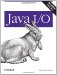# File Viewer, Part 4

Because of the nature of filter streams, it is relatively straightforward to add decompression services to the FileDumper program last seen in Chapter 8. Generally, you'll want to decompress a file before dumping it. Adding decompression does not require a new dump filter. Instead, it simply requires passing the file through an inflater input stream before passing it to one of the dump filters. We'll let the user choose from either gzipped or deflated files with the command-line switches -gz and -deflate. When one of these switches is seen, the appropriate inflater input stream is selected; it is an error to select both. Example 10-14, FileDumper4, demonstrates.

Example 10-14. FileDumper4

 ```import java.io.*; import java.util.zip.*; import com.elharo.io.*; public class FileDumper4 { public static final int ASC = 0; public static final int DEC = 1; public static final int HEX = 2; public static final int SHORT = 3; public static final int INT = 4; public static final int LONG = 5; public static final int FLOAT = 6; public static final int DOUBLE = 7; public static void main(String[] args) { if (args.length < 1) { System.err.println("Usage: java FileDumper4 [-ahdsilfx] [-little]"+ "[-gzip|-deflated] file1..."); } boolean bigEndian = true; int firstFile = 0; int mode = ASC; boolean deflated = false; boolean gzipped = false; // Process command-line switches. for (firstFile = 0; firstFile < args.length; firstFile++) { if (!args[firstFile].startsWith("-")) break; if (args[firstFile].equals("-h")) mode = HEX; else if (args[firstFile].equals("-d")) mode = DEC; else if (args[firstFile].equals("-s")) mode = SHORT; else if (args[firstFile].equals("-i")) mode = INT; else if (args[firstFile].equals("-l")) mode = LONG; else if (args[firstFile].equals("-f")) mode = FLOAT; else if (args[firstFile].equals("-x")) mode = DOUBLE; else if (args[firstFile].equals("-little")) bigEndian = false; else if (args[firstFile].equals("-deflated") && !gzipped) deflated = true; else if (args[firstFile].equals("-gzip") && !deflated) gzipped = true; } for (int i = firstFile; i < args.length; i++) { try { InputStream in = new FileInputStream(args[i]); dump(in, System.out, mode, bigEndian, deflated, gzipped); if (i < args.length-1) { // more files to dump System.out.println( ); System.out.println("--------------------------------------"); System.out.println( ); } } catch (Exception e) { System.err.println(e); e.printStackTrace( ); } } } public static void dump(InputStream in, OutputStream out, int mode, boolean bigEndian, boolean deflated, boolean gzipped) throws IOException { // The reference variable in may point to several different objects // within the space of the next few lines. We can attach // more filters here to do decompression, decryption, and more. if (deflated) { in = new InflaterInputStream(in); } else if (gzipped) { in = new GZIPInputStream(in); } if (bigEndian) { DataInputStream din = new DataInputStream(in); switch (mode) { case HEX: in = new HexFilter(in); break; case DEC: in = new DecimalFilter(in); break; case INT: in = new IntFilter(din); break; case SHORT: in = new ShortFilter(din); break; case LONG: in = new LongFilter(din); break; case DOUBLE: in = new DoubleFilter(din); break; case FLOAT: in = new FloatFilter(din); break; default: } } else { LittleEndianInputStream lin = new LittleEndianInputStream(in); switch (mode) { case HEX: in = new HexFilter(in); break; case DEC: in = new DecimalFilter(in); break; case INT: in = new LEIntFilter(lin); break; case SHORT: in = new LEShortFilter(lin); break; case LONG: in = new LELongFilter(lin); break; case DOUBLE: in = new LEDoubleFilter(lin); break; case FLOAT: in = new LEFloatFilter(lin); break; default: } } StreamCopier.copy(in, out); in.close( ); } } ```

Note how little I had to change to add support for compressed files. I simply imported one package and added a couple of command-line switches and six lines of code (which could easily have been two) to test for the command-line arguments and add one more filter stream to the chain. Zip and JAR files would not be hard to support either. You'd just have to iterate through the entries in the archive and dump each entry onto System.out. That's left as an exercise for the reader.Java I/O
ISBN: 0596527500
EAN: 2147483647
Year: 2004
Pages: 244